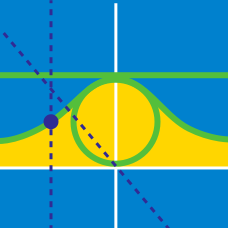Calculus

# Parametric Equations - Volume

Find the volume of the three dimensional shape obtained by revolving the curve described by the parametric equations below about the $x$-axis from $t=0$ to $\displaystyle t=\frac{\pi}{2}:$ \begin{aligned} x(t) &= 3 \cos t \\ y(t) &= 4 \sin t. \end{aligned}

Find the volume of the three dimensional shape obtained by revolving the curve described by the parametric equations below about the $y$-axis from $t=1$ to $t=5:$ \begin{aligned} x(t) &= 15 t^2 \\ y(t) &= 6 t. \end{aligned}

Find the volume of the three dimensional shape obtained by the following equations from $r=0$ to $r= 1,$ from $t=0$ to $t= 3,$ and $u = 0$ to $u = 2 \pi:$ \begin{aligned} x(r,t,u) &= 3 t \\ y(r,t,u) &= r\sqrt{ t^2+ 6t } \cos u \\ z(r,t,u) &= r\sqrt{ t^2 + 6t } \sin u. \end{aligned}

Find the volume of the three dimensional shape obtained by the following equations from $t=-5$ to $t= 5,$ $u=-4$ to $u= 4,$ and $w = 0$ to $w = 1:$ \begin{aligned} x(t,u,w) &= (1-w)t \\ y(t,u,w) &= (1-w)u \\ z(t,u,w) &= 9 w. \end{aligned}

Find the volume of the three dimensional shape obtained by the following equations from $r = 0$ to $r = 1,$ from $t=0$ to $t=3 \pi ,$ and $u = 0$ to $u = 2 \pi:$ \begin{aligned} x(r,t,u) &= 9 rt ( \cos u ) \\ y(r,t,u) &= 2 rt ( \sin u) \\ z(r,t,u) &= 4t. \end{aligned}

×